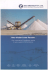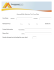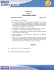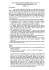# Class 10 - Sample Question Paper (Mathematics) I – SA-I

## Transcription

Class 10 - Sample Question Paper (Mathematics) I – SA-I
```Class 10 - Sample Question Paper (Mathematics) I – SA-I
Time allowed: 3 hours Maximum marks: 80
General Instructions:
(i)
All questions are compulsory.
(ii)
The question paper consists of 34 questions divided into four sections – A, B, C
and D.
(iii) Section A contains 10 questions of 1 mark each, which are multiple choice type
questions, section B contains 8 questions of 2 marks each, section C contains 10
questions of 3 marks each and section D contains 6 questions of 4 marks each.
(iv) There is no overall choice in the paper.
(v)
Use of calculators is not permitted.
Section – A
Question numbers from 1 to 10 are of one mark each.
1. Let
be a rational number, such that the prime factorization of q is of the form
2n5m, where n, m are non-negative integers. Then the decimal expansion of x is
a) Terminating
b) Non-terminating recurring
c) Non-terminating non recurring
d) None
2. Given positive integers a and b, there exist unique integers q and r satisfying a = bq
+ r, where r satisfies
a) 0 <r < b
b) 0 < r ≤ b
c) 0 ≤r < b
d) 0 ≤r ≤ b
3. The sum of the zeroes of the quadratic polynomial
is
a) –
b)
c) –
d)
Page 1
4. The lines
a) Intersecting lines
b) Parallel lines
c) Coincident lines
d) Perpendicular lines
are
5. The sides of two similar triangles are in the ratio
will be
a) 2:5
b) 4:25
c) 25:4
d) 5:2
then the ratio of their areas
6. A ladder 13 m long is placed against a vertical wall of height 12m. The distance
between the foot of the ladder and the wall is
a) 5 m
b) 10 m
c) 7 m
d) 8 m
7. If
a)
b)
c)
d)
where 2A is an acute angle then
8.
a) 1
b) 0
c)
d)
9.
a)
b)
c)
d)
Page 2
10. If the mode and median of a data are 27.8 and 26.7 then its mean is
a) 26.51
b) 27.15
c) 26.15
d) 27.51
Section – B
Question numbers 11 to 18 carry 2 marks each.
11. Given that HCF (306, 657) = 9, find LCM (306, 657).
12. Find the zeroes of the quadratic polynomial,
relationship between the zeroes and the coefficients.
13. Solve the equations
and verify the
using substitution method.
14. The diagonals of a quadrilateral intersect each other at O such that
. Show
that ABCD is a trapezium.
15. A vertical pole of length 6 m casts a shadow 4 m long on the ground and at the same
time a tower casts a shadow 28 m long. Find the height of the tower.
16. Prove that
17. Write the formula to find the median for grouped data and explain each term.
18. Find the mode of the following data:
Class
Frequency
0–9
6
10 – 19
9
20 – 29
14
30 – 39
21
40 – 49
16
50 – 59
8
Section – C
Question numbers 19 to 28 carry 3 marks each.
19. U Eu l d’ d v
l mm
h w h h qu r
of the form 3m or 3m + 1 for some integer m.
20. Show that √
f
y positive integer is either
is irrational.
Page 3
21. Divide
by
and verify the division algorithm.
22. Solve
23. State and prove basic proportionality theorem.
24. In rhombus ABCD, prove that
25. Prove that
26. Evaluate
27. Consider the following distribution of daily wages of 50 workers of a factory. Find
the mean daily wages of the workers of the factory by using an appropriate method.
Daily wages
100 – 150 150 – 200 200 – 250 250 – 300 300 – 350
Number of workers
10
15
22
17
6
28. Find the median weight of the following students shown in the distribution table.
Weight
35 – 40
40 – 45
45 – 50
50 – 55
55 – 60
60 – 65
No.
of
2
8
14
21
4
1
Students
Section – D
Question numbers 29 to 34 carry 4 marks each.
29. Obtain all the zeroes of the polynomial
if two of its
zeroes are √ and √ .
30. A boat goes 30 km upstream and 44 km downstream in 10 hours. In 13 hours, it can
go 40 km upstream and 55 km down-stream. Determine the speed of the stream and
that of the boat in still water.
31. BL and CM are medians of a
right angled at . Prove that
Page 4
32. If
then show that
33. Prove that
.
.
34. The mean of the distribution of daily wages of 50 workers of a factory is 145.2, find
the missing frequencies.
Daily Wages (in Rs)
100 – 120 120 – 140 140 – 160 160 – 180 180 – 200
Number of Workers
12
8
10
www.learnnext.com/sample-papers.htm
Page 5
Page 6
```

### Class 10 - Sample Question Paper (Mathematics) II – SA-I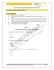### A golden opportunity to A golden opportunity to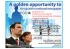### Class 9 - Sample Question Paper (Mathematics) I – SA-I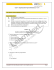### Sample Test Paper – 20 Marks Auditing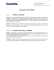### Summative Assessment-I Topper Sample Paper - 3 MATHEMATICS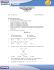### Security Settings For Windows 7 and Above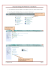### Dear Customer, HP India promotes its wide range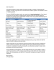### class-10 - LK Singhania Education Centre, Gotan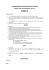### Imperium Technology Solutions (P) Ltd.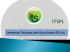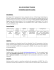### Final New Corporate overview - PRINTING.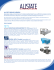### Subject – Mathematics Sample Paper – 2012 Class – X### 2013 dt 04.07.2013 on th - the official website of Bangalore Customs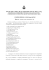### Summit Agenda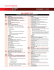### Stall Booking Form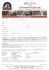### Time: 3 to 3 ½ hours M.M.:90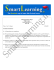### Class 10 Holiday Home Work - dav public school, rajinder nagar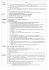### Catalog - Zeus Engitech Pvt. Ltd.